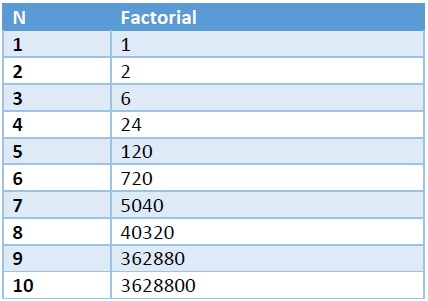# Find last two digits of sum of N factorials using C++.

C++Server Side ProgrammingProgramming

Here we will see how to get the last two digits. The unit place digit and the tens place digit of the sum of N factorials. So if N = 4, it will be 1! + 2! + 3! + 4! = 33. so unit place is 3 and ten place is 3. The result will be 33.

If we see this clearly, then as the factorials of N > 5, the unit place is 0, so after 5, it will not contribute to change the unit place. And after N > 10, the ten places will remain 0. For N = 10 and more, it will be 00. We can make a chart for N = 1 to 10 of factorial numbers.We can solve this problem using these steps −

• If the value of n is less than 10, then (1! + 2! + … + n!) mod 10
• otherwise when value of n is greater or equal to 10, then (1! + 2! + … + 10!) mod 10 = 13

## Example

#include<iostream>
#include<cmath>
using namespace std;
int getTenAndUnitPlace(long long N) {
if (N <= 10) {
long long ans = 0, factorial = 1;
for (int i = 1; i <= N; i++) {
factorial = factorial * i;
ans += factorial;
}
return ans % 100;
}
return 13;
}
int main() {
for(long long i = 1; i<15; i++){
cout << "Ten and Unit place value of sum of factorials when N = "<<i<<" is: " <<getTenAndUnitPlace(i) << endl;
}
}

## Output

Ten and Unit place value of sum of factorials when N = 1 is: 1
Ten and Unit place value of sum of factorials when N = 2 is: 3
Ten and Unit place value of sum of factorials when N = 3 is: 9
Ten and Unit place value of sum of factorials when N = 4 is: 33
Ten and Unit place value of sum of factorials when N = 5 is: 53
Ten and Unit place value of sum of factorials when N = 6 is: 73
Ten and Unit place value of sum of factorials when N = 7 is: 13
Ten and Unit place value of sum of factorials when N = 8 is: 33
Ten and Unit place value of sum of factorials when N = 9 is: 13
Ten and Unit place value of sum of factorials when N = 10 is: 13
Ten and Unit place value of sum of factorials when N = 11 is: 13
Ten and Unit place value of sum of factorials when N = 12 is: 13
Ten and Unit place value of sum of factorials when N = 13 is: 13
Ten and Unit place value of sum of factorials when N = 14 is: 13